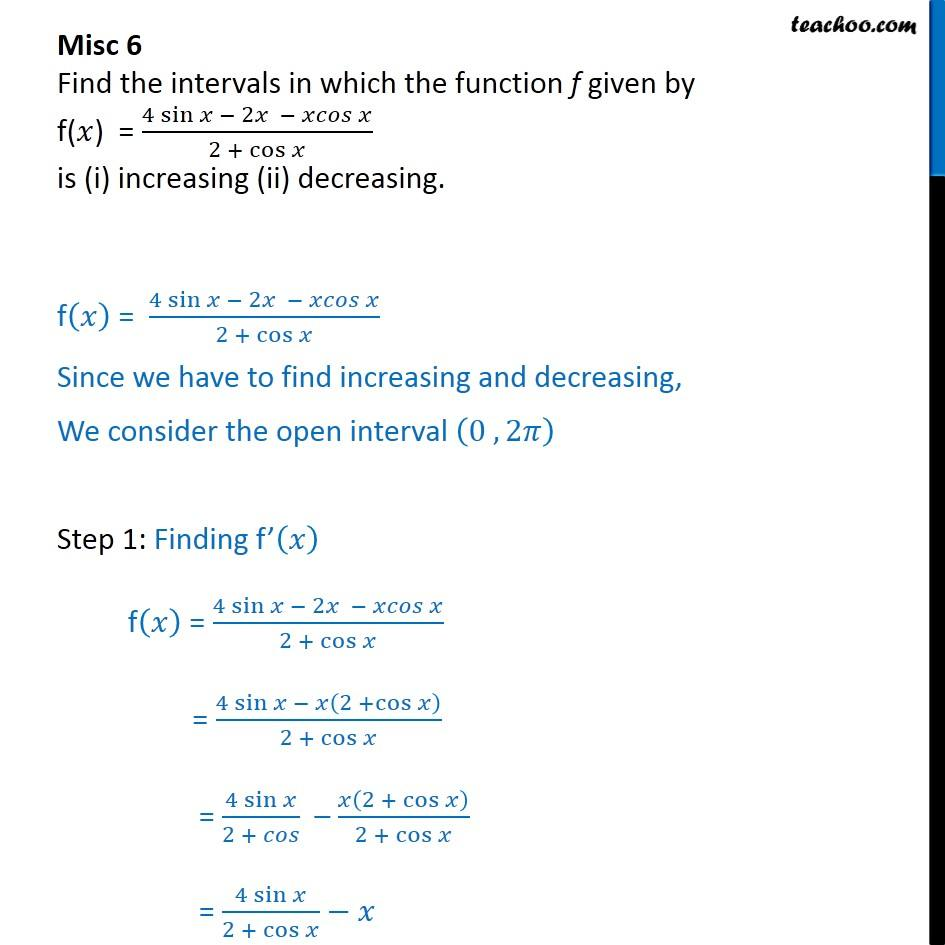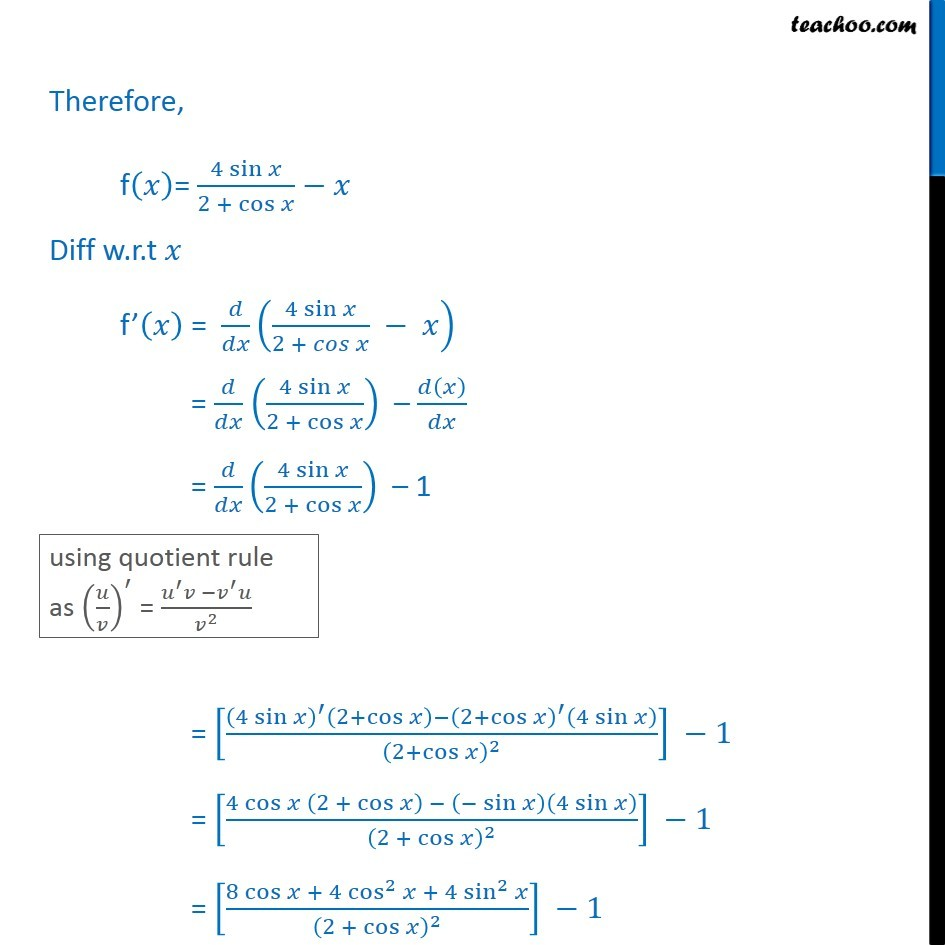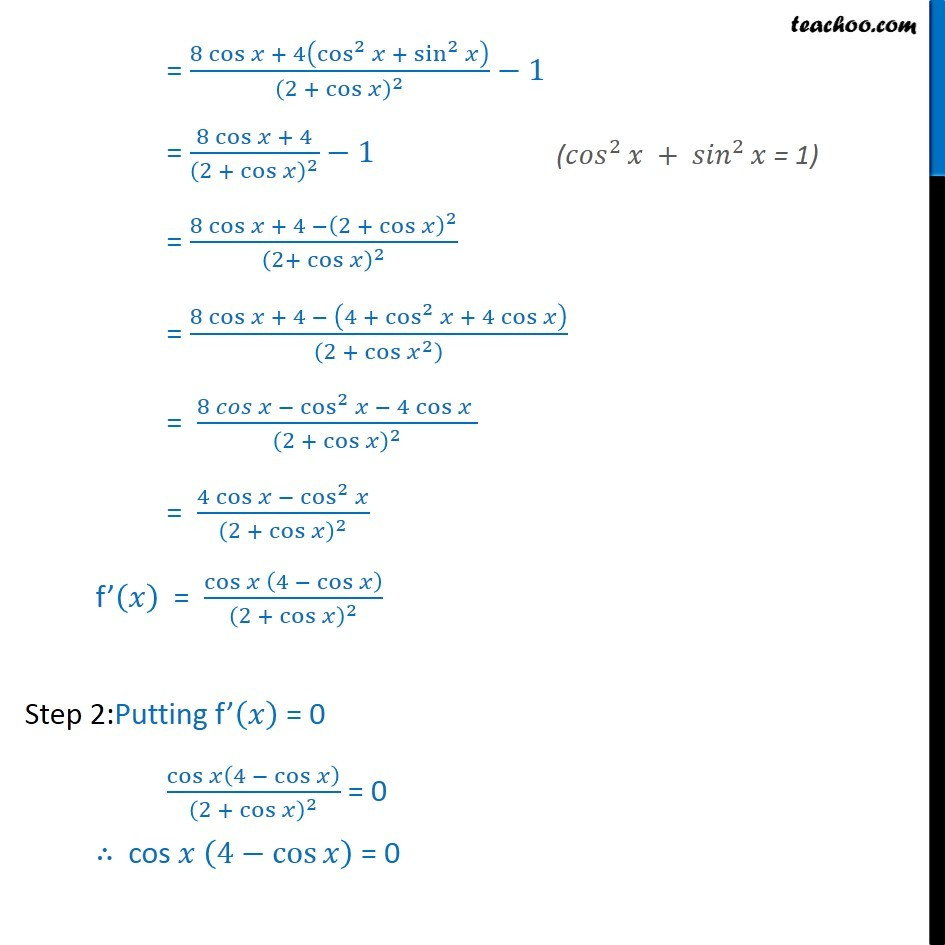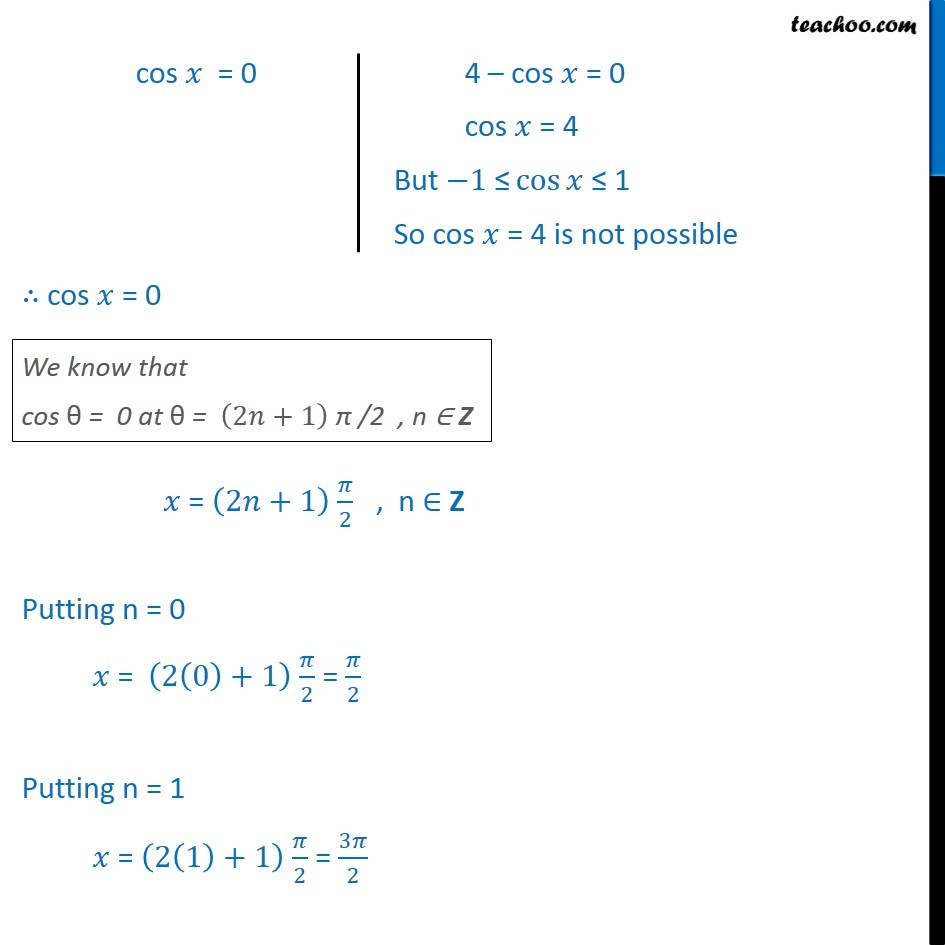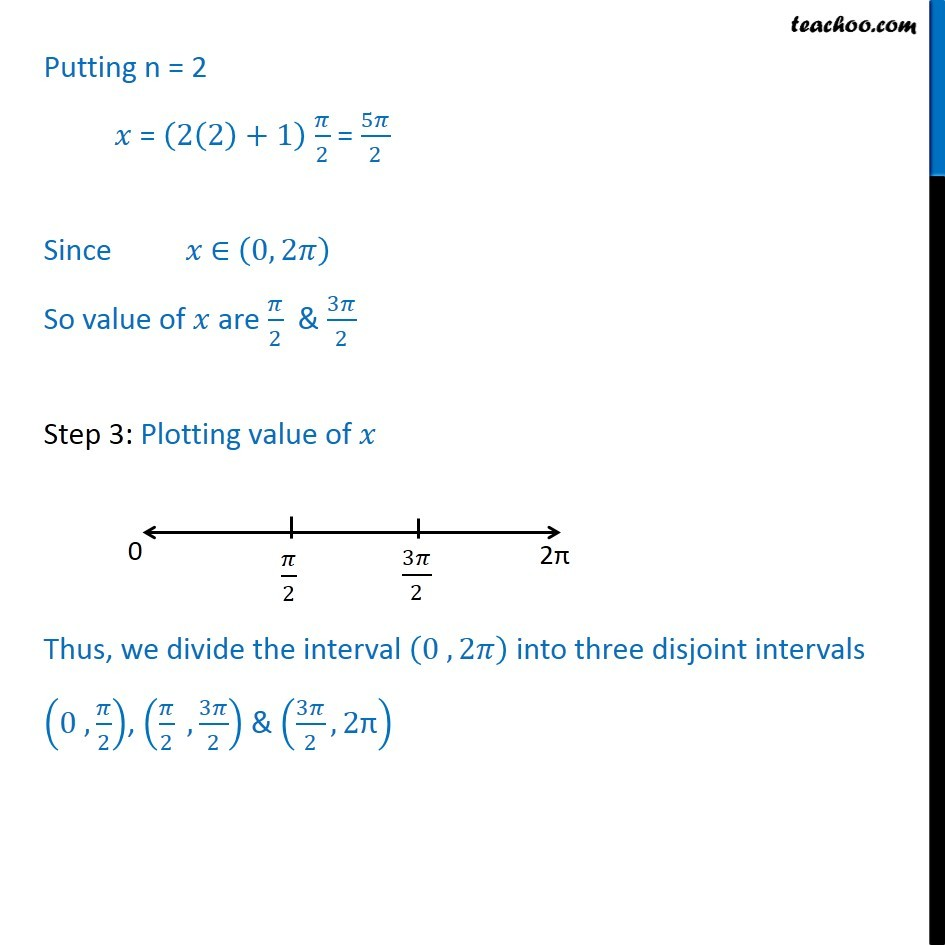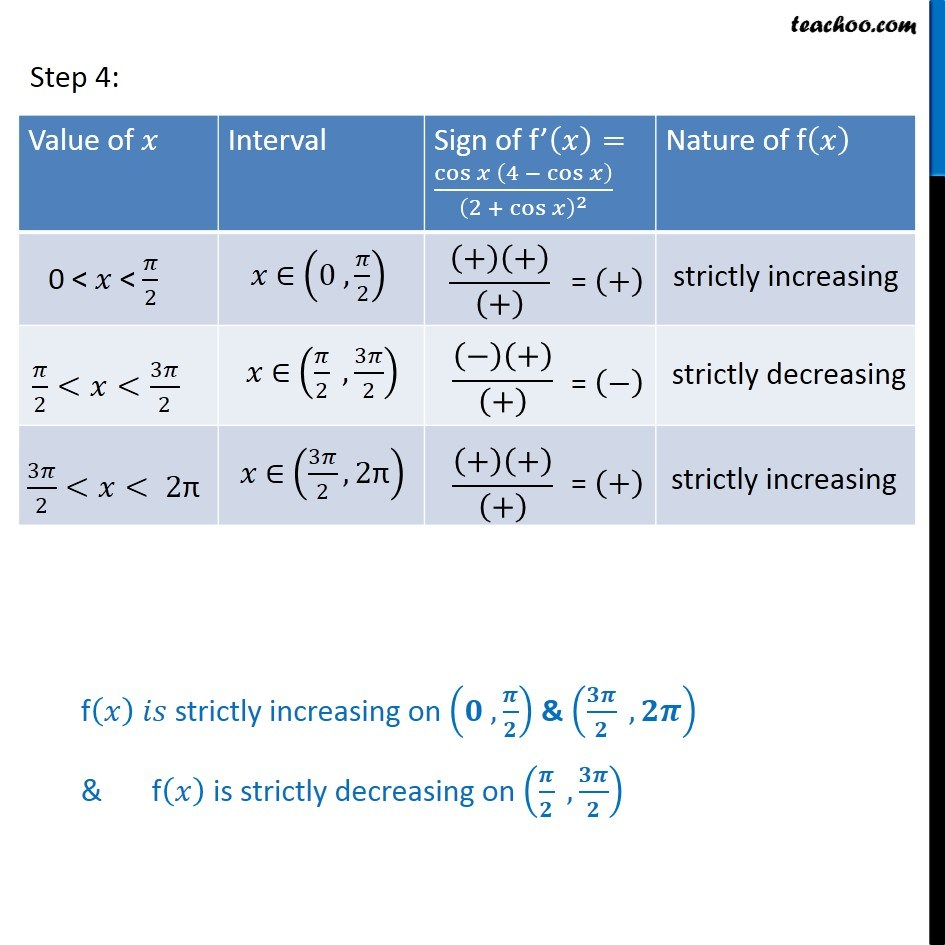1. Chapter 6 Class 12 Application of Derivatives
2. Serial order wise
3. Miscellaneous

Transcript

Misc 6 Find the intervals in which the function f given by f(𝑥) = (4 sin⁡〖𝑥 − 2𝑥 − 𝑥𝑐𝑜𝑠 𝑥〗)/(2 + cos⁡𝑥 ) is (i) increasing (ii) decreasing. f(𝑥) = (4 sin⁡〖𝑥 − 2𝑥 − 𝑥𝑐𝑜𝑠 𝑥〗)/(2 + cos⁡𝑥 ) Since we have to find increasing and decreasing, We consider the open interval (0 , 2𝜋) Step 1: Finding f’(𝑥) f(𝑥) = (4 sin⁡〖𝑥 − 2𝑥 − 𝑥𝑐𝑜𝑠 𝑥〗)/(2 + cos⁡𝑥 ) = (4 sin⁡〖𝑥 − 𝑥(2 +cos⁡𝑥 )〗)/(2 + cos⁡𝑥 ) = (4 sin⁡𝑥)/(2 + 𝑐𝑜𝑠) – 𝑥(2 + cos⁡𝑥 )/(2 + cos⁡𝑥 ) = (4 sin⁡𝑥)/(2 + cos⁡𝑥 )−𝑥 Therefore, f(𝑥)= (4 sin⁡𝑥)/(2 + cos⁡𝑥 )−𝑥 Diff w.r.t 𝑥 f’(𝑥) = 𝑑/𝑑𝑥 ((4 sin⁡𝑥)/(2 + 𝑐𝑜𝑠 𝑥) − 𝑥) = 𝑑/𝑑𝑥 ((4 sin⁡𝑥)/(2 + cos⁡𝑥 )) – 𝑑(𝑥)/𝑑𝑥 = 𝑑/𝑑𝑥 ((4 sin⁡𝑥)/(2 + cos⁡𝑥 )) – 1 = [((4 sin⁡𝑥 )^′ (2+cos⁡𝑥 )−(2+cos⁡𝑥 )^′ (4 sin⁡𝑥 ))/(2+cos⁡𝑥 )^2 ] −1 = [(4 cos⁡𝑥 (2 + cos⁡𝑥 ) − (−sin⁡𝑥 )(4 sin⁡𝑥 ))/(2 + cos⁡𝑥 )^2 ] −1 = [(8 cos⁡𝑥 + 4 cos^2⁡𝑥 + 4 sin^2⁡𝑥)/(2 + cos⁡𝑥 )^2 ] −1 = (8 cos⁡𝑥 + 4(cos^2⁡𝑥 +〖 sin^2〗⁡𝑥 ))/(2 + cos⁡𝑥 )^2 −1 = (8 cos⁡𝑥 + 4)/(2 + cos⁡𝑥 )^2 −1 = (8 cos⁡𝑥 + 4 −(2 + cos⁡𝑥 )^2)/(2+ cos⁡𝑥 )^2 = (8 cos⁡〖𝑥 + 4 − (4 + cos^2⁡〖𝑥 + 4 cos⁡𝑥 〗 )〗)/((2 + 〖cos⁡𝑥〗^2 ) ) = (8 𝑐𝑜𝑠 𝑥 − cos^2⁡𝑥 − 4 cos⁡𝑥 )/(2 +〖 cos〗⁡𝑥 )^2 = (4 cos⁡〖𝑥 −〖 cos〗^2⁡𝑥 〗)/(2 + cos⁡𝑥 )^2 f’(𝑥) = cos⁡〖𝑥 (4 − cos⁡𝑥 )〗/(2 + cos⁡𝑥 )^2 Step 2:Putting f’(𝑥) = 0 cos⁡𝑥(4 − cos⁡𝑥 )/(2 + cos⁡𝑥 )^2 = 0 ∴ cos 𝑥 (4−cos⁡𝑥 ) = 0 ∴ cos 𝑥 = 0 𝑥 = (2𝑛+1) 𝜋/2 , n ∈ Z Putting n = 0 𝑥 = (2(0)+1) 𝜋/2 = 𝜋/2 Putting n = 1 𝑥 = (2(1)+1) 𝜋/2 = 3𝜋/2 cos 𝑥 = 0 4 – cos 𝑥 = 0 cos 𝑥 = 4 But −1" ≤" cos⁡𝑥 ≤ 1 So cos 𝑥 = 4 is not possible ∴ cos 𝑥 = 0 We know that cos θ = 0 at θ = (2𝑛+1) π /2 , n ∈ Z 𝑥 = (2𝑛+1) 𝜋/2 , n ∈ Z Putting n = 0 𝑥 = (2(0)+1) 𝜋/2 = 𝜋/2 Putting n = 1 𝑥 = (2(1)+1) 𝜋/2 = 3𝜋/2 Putting n = 2 𝑥 = (2(2)+1) 𝜋/2 = 5𝜋/2 Since 𝑥 ∈ (0, 2𝜋) So value of 𝑥 are 𝜋/2 & 3𝜋/2 Step 3: Plotting value of 𝑥 Thus, we divide the interval (0 , 2𝜋) into three disjoint intervals (0 ,𝜋/2), (𝜋/2 ,3𝜋/2) & (3𝜋/2,2"π" ) f(𝑥) 𝑖𝑠 strictly increasing on (𝟎 , 𝝅/𝟐) & (𝟑𝝅/𝟐 , 𝟐𝝅) & f(𝑥) is strictly decreasing on (𝝅/𝟐 ,𝟑𝝅/𝟐)

Miscellaneous